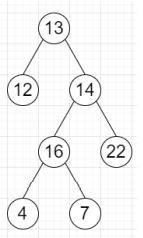# Program to find leftmost deepest node of a tree in Python

Suppose we have a binary tree; we have to find the value of the deepest node. If there are more than one deepest node, then return the leftmost deepest node.

So, if the input is likethen the output will be 4 as 4 and 7 are deepest but 4 is left most.

To solve this, we will follow these steps −

• queue := a queue with one node root.

• left_max := value of root

• while size of queue > 0, do

• level_size := size of queue

• for i in range 0 to level_size, do

• temp := first element from queue and delete it

• if i is same as 0, then

• left_max := value of temp

• if left of temp is non-zero, then

• insert left of temp at the end of queue

• if right of temp is not null, then

• insert right of temp at the end of queue

• return left_max

Let us see the following implementation to get better understanding −

## Example

Live Demo

class TreeNode:
def __init__(self, value):
self.val = value
self.left = None
self.right = None

class Solution:
def solve(self, root):
queue = [root]
left_max = root.val
while len(queue) > 0:
level_size = len(queue)
for i in range(level_size):
temp = queue.pop(0)
if i == 0:
left_max = temp.val
if temp.left:
queue.append(temp.left)
if temp.right:
queue.append(temp.right)
return left_max
ob = Solution()
root = TreeNode(13)
root.left = TreeNode(12)
root.right = TreeNode(14)
root.right.left = TreeNode(16)
root.right.right = TreeNode(22)
root.right.left.left = TreeNode(4)
root.right.left.right = TreeNode(7)
print(ob.solve(root))

## Input

root = TreeNode(13)
root.left = TreeNode(12)
root.right = TreeNode(14)
root.right.left = TreeNode(16)
root.right.right = TreeNode(22)
root.right.left.left = TreeNode(4)
root.right.left.right = TreeNode(7)



## Output

4